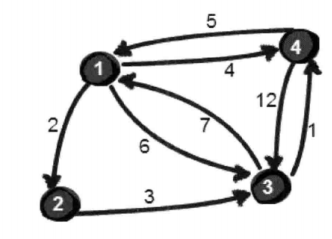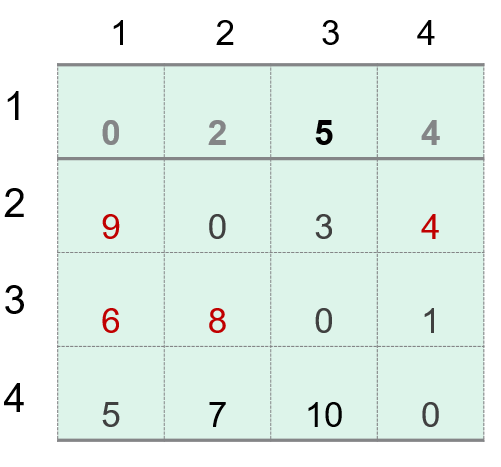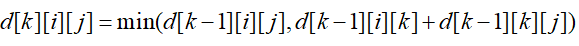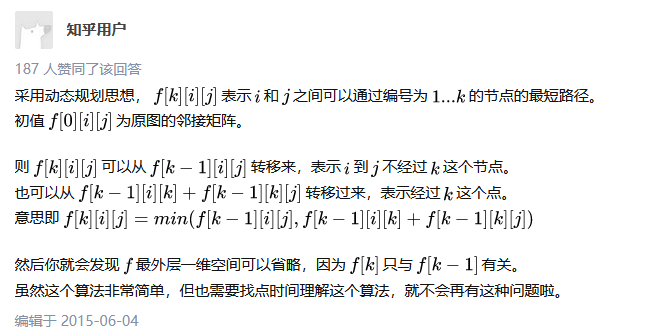• C++Floyd算法求最短路径问题
千次阅读
2022-03-19 20:41:16

## Floyd算法

Floyd算法（Floyd-Warshall algorithm）又称为弗洛伊德算法、插点法，是解决给定的加权图中顶点间的最短路径的一种算法，可以正确处理有向图或负权的最短路径问题，同时也被用于计算有向图的传递闭包。该算法名称以创始人之一、1978年图灵奖获得者、斯坦福大学计算机科学系教授罗伯特·弗洛伊德命名。

优点：容易理解，代码简单

缺点：时间复杂度比较高

# 代码

//n 为二维数组 a 的长度
for(int i=1;i<=n;i++)
for(int j=1;j<=n;j++)
for(int k=1;k<=n;k++){
if(a[i][j]>a[i][k]+a[k][j]){
a[i][j]=a[i][k]+a[k][j];
}
}c++
更多相关内容
• 主要为大家详细介绍了Java实现Floyd算法求最短路径，具有一定的参考价值，感兴趣的小伙伴们可以参考一下
• 本文实例讲述了Python基于Floyd算法求解最短路径距离问题。分享给大家供大家参考，具体如下： Floyd算法和Dijkstra算法，相信大家都不陌生，在最短路径距离的求解中应该算得上是最为基础和经典的两个算法了，今天就...
• floyd算法求最短路径的权值，java实现的
• Floyd算法用来解决全源最短路径问题，即对给定的图G(V,E)，任意两点u，v之间的最短路径长度。 ●Floyd算法基于这样一个事实：如果存在顶点k，使得以k作为中介点时顶点i和顶点j的当前最短距离缩短，则使用顶点k...

# Floyd算法

Floyd 算法用来解决全源最短路径问题，即对给定的图 G(V,E) ，求任意两点 u v 之间的最短路径长度。
Floyd 算法基于这样一个事实：如果存在顶点 k ，使得以 k 作为中介点时顶点 i 和顶点 j 的当前最短距离缩短，则使用顶点 k 作为顶点 i 和顶点 j 的中介点，即当 dis[ i ][k]+dis[k][j]<dis[ i ][j] 时，令 dis[ i ][j]= dis[ i][k]+dis[k][j] (其 中dis[i ][j] 表示从顶点 i 到顶点 j 的最短距离）。

### 图:### 运算结果 :### 代码 :

#include<iostream>
#include<vector>
using namespace std;
const int INF=99999;
class Graph {
public:
int N,E;
int type;
vector<vector<int> > g;
Graph(int n=0,int e=0,int type=0) {
N=n;
E=e;
type=type;
g=vector<vector<int> >(N+1,vector<int>(N+1,INF));
}
g[v][d]=w;
if(type==1) {
g[d][v]=w;
}
}
print() {
for(int i=1; i<=N; i++) {
for(int j=1; j<=N; j++) {
cout<<g[i][j]<<" ";
}
cout<<endl;
}
}

};

void floyd(Graph g) {
for(int k=1; k<=g.N; k++) {//中介点
for(int i=1; i<=g.N; i++) {//起点
for(int j=1; j<=g.N; j++) {//终点
if(g.g[i][k]+g.g[k][j]<g.g[i][j])
g.g[i][j]=g.g[i][k]+g.g[k][j];
}
}
}
for(int i=1; i<=g.N; i++) {
g.g[i][i]=0;
}
g.print();
}
int main(void) {
Graph g(4,8,0);
floyd(g);
return 0;
}

展开全文• 算法与数据结构的结课课程的报告。参考文章加自己提炼。求解几个点之间的最短距离的算法。c++源码在vs2019可以实现，也比较好容易理解。希望有所帮助。没有要积分，因为我也是参考别人的。
• Floyd算法用来解决多源最短路径，即可得到图中任意两个结点之间的最短路径。 该算法基于动态规划的思想。假设有n个节点，目标是节点i到达节点j的最短路径。 每次的时候，都将节点i到节点j经过k个节点到达最短...

# 算法介绍

Floyd算法用来解决多源最短路径，即可得到图中任意两个结点之间的最短路径。

该算法基于动态规划的思想。假设有n个节点，目标是求节点i到达节点j的最短路径。

每次求的时候，都将节点i到节点j经过k个节点到达最短路径状态记录下来，即d[k][i][j]，意为在状态k下，从节点i经过编号为1、2…k到节点j时路径最短。

当要找下一个节点的最短路径时，使用之前已经记录的状态进行对比，可得到新的状态转移方程便可以此状态转移方式，遍历所有点之间的情况构造最短路径算法。

# 题目描述

给定一个 n 个点 m条边的有向图，图中可能存在重边和自环，边权可能为负数。

再给定 k个询问，每个询问包含两个整数 x 和 y，表示查询从点 x 到点 y的最短距离，如果路径不存在，则输出 impossible

数据保证图中不存在负权回路。

输入格式

一行包含三个整数 n,m,k。
接下来 m行，每行包含三个整数 x,y,z，表示存在一条从点 x 到点 y 的有向边，边长为 z。
接下来 k行，每行包含两个整数 x,y，表示询问点 x 到点 y的最短距离。

输出格式

共 k行，每行输出一个整数，表示询问的结果，若询问两点间不存在路径，则输出 impossible。

数据范围

1≤n≤200,
1≤k≤n2
1≤m≤20000,
图中涉及边长绝对值均不超过 10000。

输入样例：

3 3 2
1 2 1
2 3 2
1 3 1
2 1
1 3

输出样例：

impossible
1

# 题目分析

算法的核心是对所有点的遍历部分，使用三层for循环进行实现。

for(k)			// k为中转点，起始点为1
for(i)		// i为起始点，起始点为1
for(j)	// j为目标点，起始点为1
d[i][j] = min(d[i][j], d[i][k] + d[k][j])


在这里有一个重要的地方，三层for循环的结构虽然简单，但每层for循环的含义必须要按顺序设计，否则将会得到错误的答案。Floyd算法在代码实现上虽然简单但并不是很多人都真正的懂这个算法，原因就在于对于for循环的理解。

这里有两个很赞的答案：

（1）从数学角度（2）从程序设计角度参考资料：
Floyd算法为什么把k放在最外层

为什么Floyd算法中k必须放在最外层

# 算法实现

#include <stdio.h>

const int N = 210, INF = 1e9;       //由于题中权重不超过1e4，因此取INF为1e9（2倍的指数再多一位）
int d[N][N];    // 邻接矩阵

int min(int a, int b)   {   return a < b ? a : b;   }

void floyd(int n) {
for(int k = 1; k <= n; k++)
for(int i = 1; i <= n; i++)
for(int j = 1; j <= n; j++)
d[i][j] = min(d[i][j], d[i][k] + d[k][j]);
}

int main(){
int n, m, q;        scanf("%d%d%d", &n, &m, &q);
int x, y, z;        // 一对点和权重
// 初始化邻接矩阵为无穷大
for(int i = 1; i <= n; i++)
for(int j = 1; j <= n; j++)
if(i != j)   d[i][j] = INF;

// 获取权重最小的那条边
while(m--){
scanf("%d%d%d", &x, &y, &z);
d[x][y] = min(d[x][y], z);
}
floyd(n);

// 查询q对最短路径
while(q--){
scanf("%d%d", &x, &y);
if(d[x][y] > INF / 2)       // 若不存在路径则输出impossible
puts("impossible");
else
printf("%d\n", d[x][y]);
}

return 0;
}


## 无注释代码

#include <stdio.h>

const int N = 210, INF = 1e9;

int min(int a, int b)   {   return a < b ? a : b;    }

void floyd(int n, int d[][N]){
for(int k = 1; k <= n; k++)
for(int i = 1; i <= n; i++)
for(int j = 1; j <= n; j++)
d[i][j] = min(d[i][j], d[i][k] + d[k][j]);
}

int main(){
int n, m, q;
int x, y, z;
int d[N][N];
scanf("%d%d%d", &n, &m, &q);
for(int i = 1; i <= n; i++)
for(int j = 1; j <= n; j++)
if(i != j)  d[i][j] = INF;

while(m--){
scanf("%d%d%d", &x, &y, &z);
if(d[x][y] > z)     d[x][y] = z;
}
floyd(n, d);

while(q--){
scanf("%d%d", &x, &y);
if(d[x][y] > INF / 2)       puts("impossible");
else    printf("%d\n", d[x][y]);
}

return 0;
}


时间复杂度为O(n3)

展开全文动态规划 数据结构
• #include <stdio.h> #include <...//二位数组保存每对顶点中的最短路径长度 int **nextVex;//二维数组保存vi到vj最短路径上vi后续节点的下标 }ShortPath; typedef struct GRAPHMATRIX_STRU {
#include <stdio.h>
#include <stdlib.h>
#define MAX 1000
typedef struct SHORTRSTPATH_STRU
{
int size;//图中节点的个数
int**pathLen;//二位数组保存每对顶点中的最短路径长度
int **nextVex;//二维数组保存vi到vj最短路径上vi后续节点的下标
}ShortPath;
typedef struct GRAPHMATRIX_STRU
{
int size;
int **graph;
}GraphMatrix;

GraphMatrix* InitGraph(int num)
{
int i,j;
GraphMatrix *graphMatrix=(GraphMatrix*)malloc(sizeof(GraphMatrix));
graphMatrix->size=num;
graphMatrix->graph=(int**)malloc(sizeof(int*)*graphMatrix->size);
for(i=0;i<graphMatrix->size;i++)
graphMatrix->graph[i]=(int*)malloc(sizeof(int)*graphMatrix->size);
for(i=0;i<graphMatrix->size;i++)
{
for(j=0;j<graphMatrix->size;j++)
graphMatrix->graph[i][j]=MAX;

}
return graphMatrix;
}
{
int vex1,vex2,weight;
scanf("%d%d%d",&vex1,&vex2,&weight);
while(vex1!=0||vex2!=0)
{
graphMatrix->graph[vex1][vex2]=weight;
scanf("%d%d%d",&vex1,&vex2,&weight);
}

}

ShortPath*floyd(GraphMatrix*graphMatrix)
{
int i,j,vex;
ShortPath *shortPath=NULL;
shortPath=(ShortPath*)malloc(sizeof(ShortPath));
shortPath->size=graphMatrix->size;
//分配空间二维数组
shortPath->nextVex=(int**)malloc(sizeof(int*)*shortPath->size);
for(i=0;i<shortPath->size;i++)
shortPath->nextVex[i]=(int*)malloc(sizeof(int)*shortPath->size);
//分配空间二维数组
shortPath->pathLen=(int**)malloc(sizeof(int*)*shortPath->size);
for(i=0;i<shortPath->size;i++)
shortPath->pathLen[i]=(int*)malloc(sizeof(int)*shortPath->size);
//初始化
for(i=0;i<shortPath->size;i++)
{
for(j=0;j<shortPath->size;j++)
{
//为了不破坏图的原有结构，使用辅助数组进行数据的迭代
shortPath->pathLen[i][j]=graphMatrix->graph[i][j];
if(shortPath->pathLen[i][j]==MAX)
shortPath->nextVex[i][j]=-1;
else
shortPath->nextVex[i][j]=j;
}
}
//正式开始计算
for(vex=0;vex<graphMatrix->size;vex++)
{
for(i=0;i<graphMatrix->size;i++)
{
for(j=0;j<graphMatrix->size;j++)
{
if(shortPath->pathLen[i][vex]==MAX||shortPath->pathLen[vex][j]==MAX)
continue;
//路径更短，则更新
if(shortPath->pathLen[i][vex]+shortPath->pathLen[vex][j]<shortPath->pathLen[i][j])
{
shortPath->pathLen[i][j]=shortPath->pathLen[i][vex]+shortPath->pathLen[vex][j];
shortPath->nextVex[i][j]=shortPath->nextVex[i][vex];
}
}
}

}
return shortPath;
}
int main()
{
//最短路径测试
GraphMatrix*graphMatrix=NULL;
ShortPath*shortPath=NULL;
int i,j,k,e,maxdistan,min=0;
graphMatrix=InitGraph(6);
shortPath=floyd(graphMatrix);//使用floyd算法
for(i=0;i<graphMatrix->size;i++)
{

for(j=0;j<graphMatrix->size;j++)
{
if(i!=j&&shortPath->pathLen[i][j]!=MAX)
{
printf("从%d到%d的最短路径长度为%d,具体路径为",i,j,shortPath->pathLen[i][j]);
printf("%d",i);//输出起点
k=i;//从起点出发，寻找完整路径
while(k!=j)
{
k=shortPath->nextVex[k][j];
printf("->%d",k);//将途径点输出
}
printf("\n");
/*if(min<shortPath->pathLen[i][j])
{
min=shortPath->pathLen[i][j];
maxdistan[i]=min;
}
if(i==0)
{
printf("%d->%d:%d",i,j,shortPath->pathLen[i][j]);
printf("\n");
printf("%d",i);
k=i;
while(k!=j)
{
k=shortPath->nextVex[k][j];
printf("->%d",k);
}
printf("\n");
}*/
}
}
}
return 0;
}展开全文数据结构
• floyd算法(多源最短路径) python实现 例题 下图各节点最短路径 Floyd算法如下： from math import * import numpy as np #创建节点字典 set_nodes={"v1":0,"v2":1,"v3":2,"v4":3,"v5":4,"v6":5,"v7":6,"v8":7,"v9...
• 沈阳理工大学课程设计专用纸 摘 要 现实生活中许多实际问题的解决...Visual C++6.0 的控制台工程和MFC 工程分别实现基于 floyd 算法求最短路径的应用 关键词最短路径floyd 算法邻接矩阵MFC 工程 I 沈阳理工大学课程设计
• 前面讲述了利用贪心算法求解最短路径的两种算法，分别是BFS以及Dijkstra算法。接下来要介绍的这种是一种动态规划的算法——弗洛伊德算法。 用通俗的语言来描述的话，首先我们的目标是寻找从点i到点j的最短路径。从...
• 求最短路径Floyd算法实现，无向图和有向图均适用。1先区别有向图和无向图，2输入顶点数和边数并检查合法性，3输入每边的起点、终点、权重并检查合法性，并初始化邻接矩阵和路径矩阵，4调用自定义函数Floyd
• 1)每对顶点的最短路径; 2)有向图、无向图和混合图; 算法思想:  直接在图的带权邻接矩阵中用插入顶点的方法依次递推地构造出n个矩阵D(1), D(2), …, D(n), D(n)是图的距离矩阵, 同时引入一个后继点矩阵记录两点...
• 现实生活中许多实际问题的解决依赖于最短路径的应用其中比较常用的是floyd算法通过floyd算法使最短路径问题变得简单化采用图的邻接矩阵或邻接表...C++6.0的控制台工程和MFC工程分别实现基于floyd算法求最短路径的应用...
• * 1，弗洛伊德算法实现 加全权图中的 寻求最短路径可以用来求解任意一点，到其他任意一点之间的最短距离。 * 2，设置顶点vi到顶点vk的最短路径已知为Lik，顶点vk到vj的最短路径已知为Lkj，顶点vi到vj的路径为Lij，...
• 对同一场景分别进行dijkstra算法求指定节点间的最短路径floyd求任意端间最短路径。 报告中含C++代码
• Floyd算法又称为弗洛伊德算法、插点法，是一种利用动态规划的思想寻找给定的加权图中多源点之间最短路径的算法，与Dijkstra算法类似。该算法名称以创始人之一、1978年图灵奖获得者、斯坦福大学计算机科学系教授...Matlab 数据建模
• Dijkstra和Floyd算法最短路径matlab实现，通信网作业
• Floyd算法直接使用二维数组出所有顶点到所有顶点的最短路径。 D代表顶点到顶点的最短路径权值和的矩阵。 P代表对应顶点的最小路径的前驱矩阵。 以下程序在DEV C++中调试运行通过。 #include <stdio> #define ...
• floyd算法完成最短路径的选择 题目：有如图A,B,C,D,E五个节点，试问从A到D的最短路径是多少步？ 问题分析：从A到D，若用穷举法，有A-B-D, A-B-E-C-D,等，此时因为节点数少，用穷举法自然能够出，若节点数...python
•数据结构
• 在得到距离矩阵D=[dij]m*n后，通过Floyd算法快速获得D的最短距离矩阵D*。
• Floyd算法求解最短路径问题（C++实现）（源码见链接） Floyd(弗洛伊德)算法也可称其为插点算法，是一种基于动态规划思想的最短路径算法。动态规划算法将求解的分体分成若干个阶段，按顺序求解子阶段并且动态规划算法...c++ 动态规划 自动驾驶...